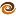# EmptyCrate

#### About Me | Contact Me | Training |ChaiScript.com | YouTube Channel

Short answer: don’t do it! Long answer: As the C++ example from the article on Real World HaskellChapter 1-2 showed:

``````#include <iostream>

bool getbool(bool b)
{
std::cout << " getbool called " << std::endl;
return b;
}

struct Boolean
{
private:
bool value;

public:
Boolean(bool t_value)
: value(t_value)
{
}

bool operator&&(const Boolean &right) const
{
return this->value && right.value;
}

bool operator||(const Boolean &right) const
{
return this->value || right.value;
}
};

Boolean getBoolean(bool b)
{
std::cout << " getBoolean called " << std::endl;
return Boolean(b);
}

int main()
{
std::cout << " Testing getbool || expecting 1 output: " << std::endl;
if (getbool(true) || getbool(false))
{
}

std::cout << " Testing getbool || expecting 2 outputs: " << std::endl;
if (getbool(false) || getbool(true))
{
}

std::cout << " Testing getbool && expecting 1 outputs: " << std::endl;
if (getbool(false) && getbool(true))
{
}

std::cout << " Testing getBoolean || expecting 2 outputs: (no shortcircuit) " << std::endl;
if (getBoolean(true) || getBoolean(false))
{
}

std::cout << " Testing getBoolean && expecting 2 outputs: (no shortcircuit)" << std::endl;
if (getBoolean(false) && getBoolean(true))
{
}
}
``````
 It is impossible to overload the && or operators in C++ and maintain the short circuiting semantics of the language. Because of this it is highly recommended that never overload these operators. So, what do we do if we want boolean tests but cannot overload the && and operators? Provide a conversion to boolean operator:
``````#include <iostream>

bool getbool(bool b)
{
std::cout << " getbool called " << std::endl;
return b;
}
struct Boolean
{
private:
bool value;

public:
Boolean(bool t_value)
: value(t_value)
{
}

operator bool() const
{
return value;
}
};

Boolean getBoolean(bool b)
{
std::cout << " getBoolean called " << std::endl;
return Boolean(b);
}

int main()
{
std::cout << " Testing getbool || expecting 1 output: " << std::endl;
if (getbool(true) || getbool(false))
{
}

std::cout << " Testing getbool || expecting 2 outputs: " << std::endl;
if (getbool(false) || getbool(true))
{
}

std::cout << " Testing getbool && expecting 1 outputs: " << std::endl;
if (getbool(false) && getbool(true))
{
}

std::cout << " Testing getBoolean || expecting 1 outputs:" << std::endl;
if (getBoolean(true) || getBoolean(false))
{
}

std::cout << " Testing getBoolean && expecting 1 outputs:" << std::endl;
if (getBoolean(false) && getBoolean(true))
{
}
}
``````

The above code works well for our case because we are providing a direct wrapper around a bool type, so our type is fully convertable to bool. However, most of the time this will not be the case and it is much better to use the Safe Bool Idiom which allows conversion to a bool equivalent without allowing for unexpected comparisons between unrelated types. The linked article does the full topic much better justice than I can.

My Videos